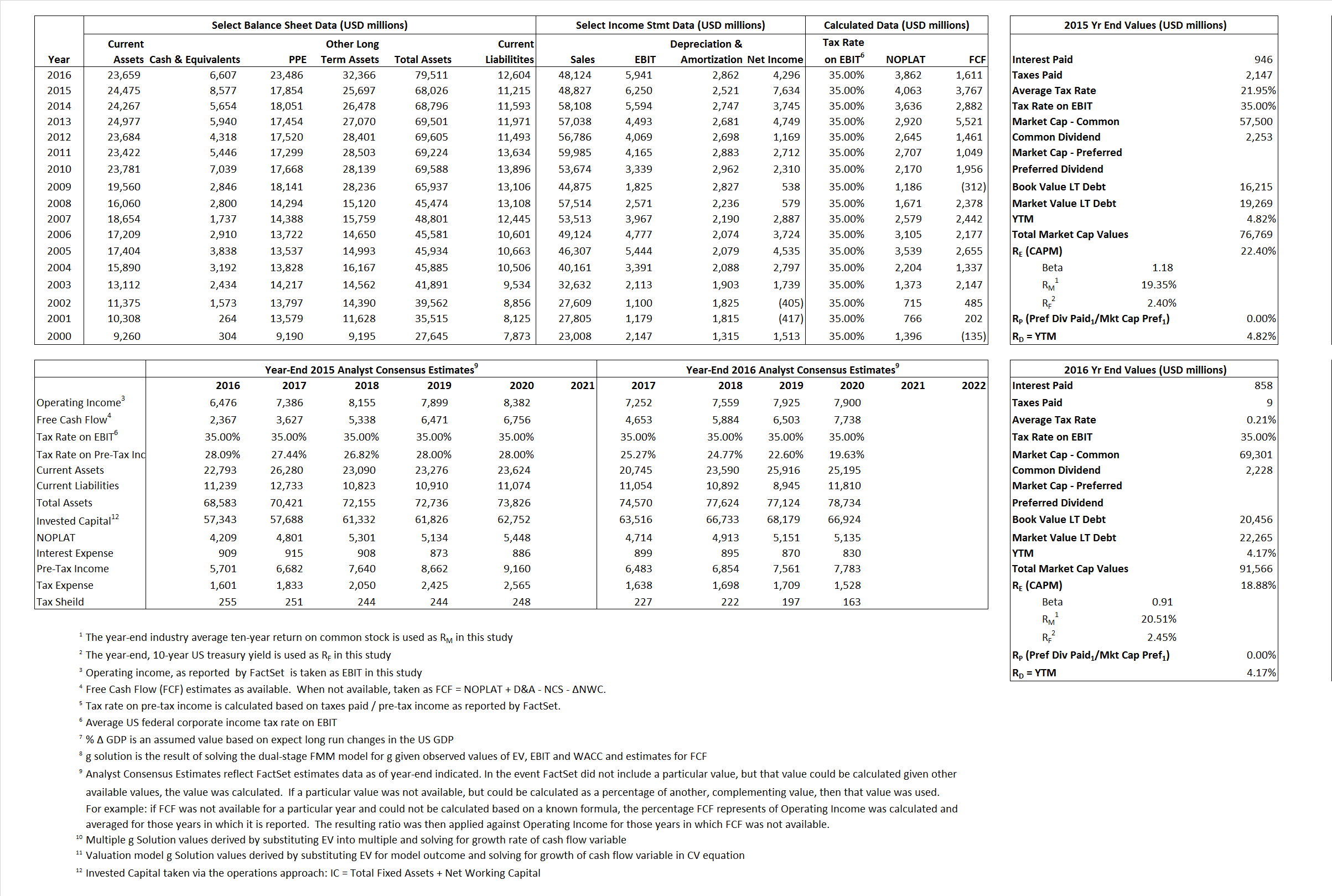# Dow Chemical Company

## Analyst Listing

The following analysts provide coverage for the subject firm as of May 2016:

 Broker Analyst Analyst Email Nomura Research Aleksey Yefremov aleksey.yefremov@nomura.com RBC Capital Markets Arun Viswanathan arun.viswanathan@rbccm.com Credit Suisse Christopher S. Parkinson christopher.parkinson@credit-suisse.com Deutsche Bank Research David Begleiter david.begleiter@db.com Susquehanna Financial Group Don Carson don.carson@sig.com Wells Fargo Securities Frank J. Mitsch frank.mitsch@wellsfargo.com Monness Crespi Hardt Herbert Hardt hhardt@mchny.com SunTrust Robinson Humphrey James Sheehan james.sheehan@suntrust.com Jefferies Laurence Alexander lalexander@jefferies.com

## Primary Input Data## Derived Input Data

### Equational Form

Net Operating Profit Less Adjusted Taxes NOPLAT  4,063  3,862$NOPLAT\, =\, EBIT\, x\, (1 \,-\, Avg \,\,Tax\,\, Rate\,\, on\,\, EBIT)$
Free Cash Flow FCF 3,767  1,611$FCF\,=NOPLAT\,+\,Non-Cash\,Expenses-\Delta NWC\,-\,NCS$
Tax Shield TS 208 2$TS\,=\,Interest\,\,Paid\,\,x\,\, Avg \,\,Tax\,\,Rate\,\, on\,\, Pre-Tax\,\, Income$
Invested Capital IC  56,811 66,907$IC\,=\,Fixed\,\,Operating\,\,Assets\,\,+\,\,Net\,\, Working\,\, Capital$
Return on Invested Capital ROIC 7.15% 5.77%$ROIC\,=\,\frac { NOPLAT }{ IC }$
Net Investment NetInv  2,129 12,958$NetInv\,=\,{ {IC}_{1}}-{{IC}_{0}}+Depreciation$
Investment Rate IR  52.41%  333.56%$IR\,=\,\frac {NetInv}{NOPLAT}$
Weighted Average Cost of Capital
WACCMarket 17.72% 15.30%$WACC\,=\,\frac { E }{ V } { R }_{ E }\,+\,\frac { P }{ V } { R }_{ P }\,+\,\frac { D }{ V } { R }_{ D }\left( 1- Avg\,\, Tax\,\,Rate\,\,on\,\,Pre-Tax\,\,Income \right)$
WACCBook  8.79%    7.21%
Enterprise value
EVMarket 68,192  84,959$EV\,=\,Market\,\,Cap\,\,Equity\,+\,\,Long\,\,Term\,\,Debt\,-\,Cash$
EVBook  65,138    83,150
Long-Run Growth
g = IR x ROIC
3.75%    19.37% Long-run growth rates of the income variable are used in the Continuing Value portion of the valuation models.
g = %$\Delta$ GDP   2.50%    2.50%
Margin from Operations M  12.80%   12.35%$M\,\,=\,\,\frac{EBIT}{SALES}$
Depreciation/Amortization Rate D  28.74%   32.51%$D\,\,=\,\,\frac{D+A}{EBITDA}$

## Valuation Multiple Outcomes

The outcomes presented in this study are the result of original input data, derived data, and synthesized inputs.

### model g solution

12/31/2015 12/31/2016 12/31/2015 12/31/2016 12/31/2015 12/31/2016

EV/SALES$\frac {EV}{Sales} \,= \,\frac{ROIC\, -\, g}{ROIC\,(WACC\,-\,g)}\,(1\,-\,T)\,(M)$

1.40  1.77  70.49%  50.64%  26.94%  22.96%

EV/EBITDA$\frac {EV}{EBITDA} \,= \,\frac{ROIC\, -\, g}{ROIC\,(WACC\,-\,g)}\,(1\,-\,T)\,(1\,-\,D)$

7.77 9.65 70.49% 50.64% 26.94% 22.96%

EV/NOPLAT$\frac {EV}{NOPLAT} \,= \,\frac{ROIC\, -\, g}{ROIC\,(WACC\,-\,g)}$

16.79 22.00 70.49% 50.64% 26.94% 22.96%

EV/FCFOPS$\frac {EV}{FCF_{OPS}} \,= \,\frac{ROIC\, -\, g}{ROIC\,(WACC\,-\,g)}\,(1\,-\,T)$

18.10 52.74 70.49% 50.64% 26.94% 22.96%

EV/EBIT$\frac {EV}{EBIT} \,= \,\frac{ROIC\, -\, g}{ROIC\,(WACC\,-\,g)}\,(1\,-\,T)$

10.91 14.30 70.49% 50.64% 26.94% 22.96%

EV/IC$\frac {EV}{IC} \,= \,\frac{ROIC\, -\, g}{WACC\,-\,g}$

1.20 1.27 70.49% 50.64% 26.94% 22.96%